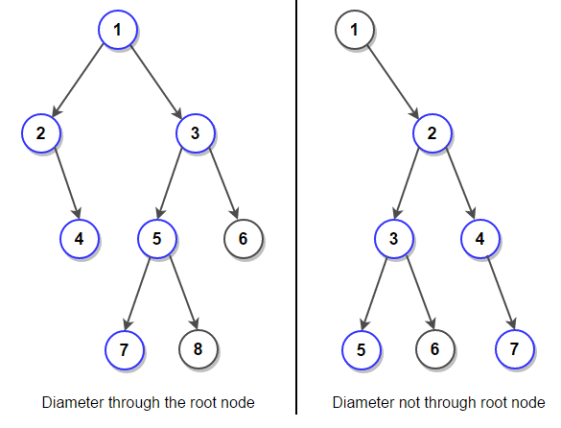You are given the reference to the root node of a binary tree. Find the diameter of the tree.

Input

The function diameterOfBinaryTree(struct node *root) only takes the reference to the root node as input.

Output

Return the diameter of the Binary Tree.

This problem has been previously asked in Amazon, Cadence India, Directi, MakeMyTrip.

The Diameter of the Binary Tree is the longest path between any two leaf nodes of the tree.

Now there are two cases -

1. The Diameter of the tree passes through the root node.
2. The Diameter of the tree does not pass through the root node.

Note: The maximum path we are talking about, starts from one leaf of the tree and ends at another leaf of the tree.In the below given solution -

1. It is firstly checked if the root node is NUll, and if it is so, the output will be simply 0.
2. Height of the left subtree is calculated.
3. Height of the right subtree is calculated.
4. If the diameter of the tree passes through the root node, then Diamter = Height in Step (2) + Height in Step (3) + 1.
5. Diameter of the tree considering only the left subtree of the current root node is calculated.
6. Diamter of the tree considering only right subtree of the current root node is calculated.
7. The maximum of the values in Step (4), Step (6), Step (7) is given as output.

The below given code is in C programming language.

```#include<stdio.h>
#include<stdlib.h>

struct node{
int data;
struct node *left;
struct node *right;
};

struct node *root = NULL;

struct node *newnode(int data){
struct node *n = (struct node *)malloc(sizeof(struct node));
n -> data = data;
return n;
}

int max(int a, int b){
if(a > b){
return a;
}
else{
return b;
}
}

int heightOfTree(struct node *root){
if(root == NULL){
return 0;
}
else{
return (1 + max(heightOfTree(root -> left), heightOfTree(root -> right)));
}
}

int diameterOfBinaryTree(struct node *root){
int left1,right1,tree_height,left2,right2;
if(root == NULL){
return 0;
}
else{
left1 = heightOfTree(root->left);
right2 = heightOfTree(root->right);
tree_height = left1 + right2 + 1;

left2 = diameterOfBinaryTree(root->left);
right2 = diameterOfBinaryTree(root->right);

return max(tree_height, max(left2,right2));
}
}

void main(){
int d;
root = newnode(10);
root -> left = newnode(20);
root -> right = newnode(30);
root -> left -> left = newnode(40);
root -> left -> right = newnode(50);
root -> left -> left -> left = newnode(60);
root -> left -> right -> left = newnode(70);
root -> left -> left -> left -> left = newnode(80);

printf("%d\n",diameterOfBinaryTree(root));
}
```